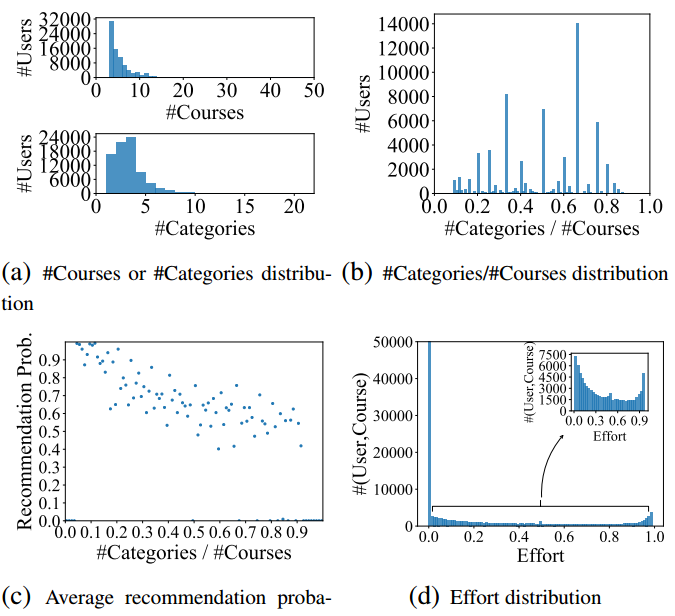## Attention Mechanism in Session Recommendation

This report focuses on the attention mechanism used in some previous session recommendation works. The conclusion is summarized in the final part: Some Intuition of Using Candidate Item as Query

## Neural Attentive Session-based Recommendation

CIKM’17, Best Paper Runner-ups Award

Problem: Session recommendation.

Gap: Previous works only consider the user’s sequential behavior in the current session, whereas the user’s main purpose in the current session is not emphasized. An example is shown in the following figure. For the session in the left part, the global recommender models the user’s whole sequential behavior to make recommendations while the proposed local recommender could capture the user’s main purpose to make recommendations.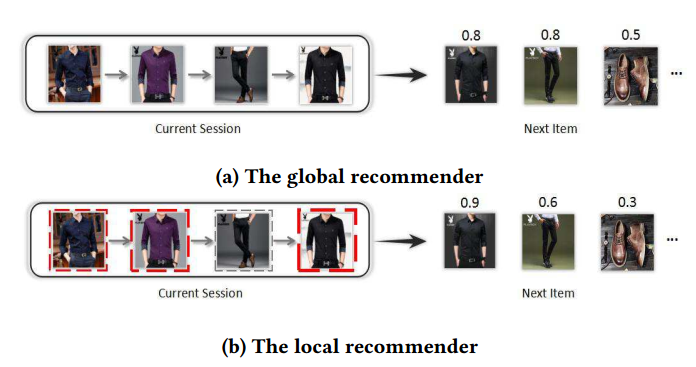Method: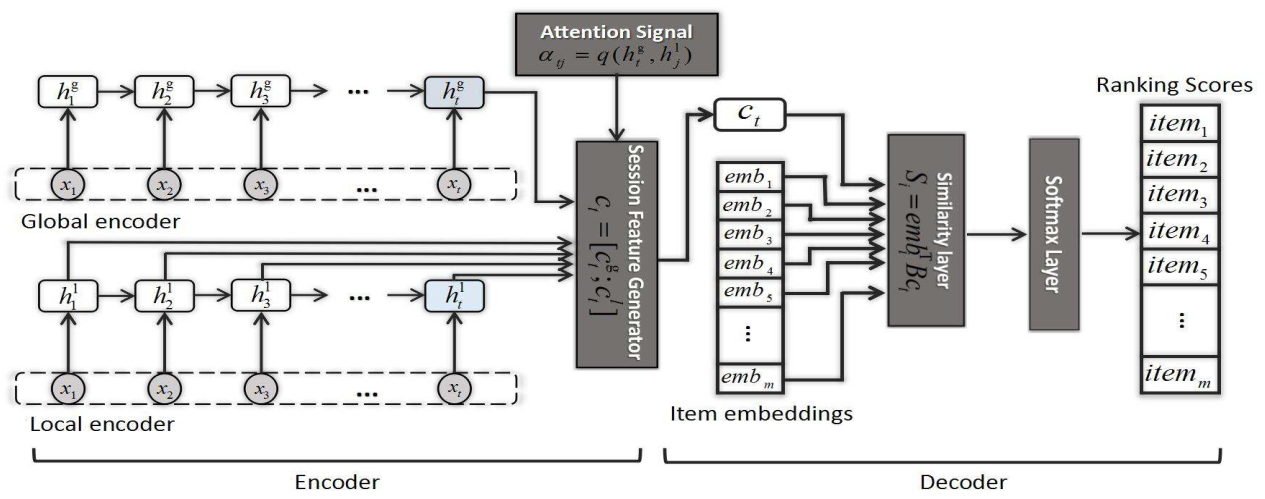As shown in the above figure, the process is summarized as

1. In the upper left part, a GRU based encoder is used to encode the session of a user. The output state is denoted as $h_t^g$.
2. In the lower left part, another GRU is used to encode the session of the user. The outputs are a sequence of hidden states, denoted as ${h_1^l, h_2^l, …,h_t^l}$.
3. In the middle, the attention mechanism is utilized to generate the preference of the current user. The attention point or query key is $h_t^g$ and the memory pool is ${h_1^l, h_2^l, …,h_t^l}$.
4. In the right part, the score for each candidate item $i$ is calculated as $S_i = emb_i^TBc_t$ where $B$ is parameter. And then a Softmax Layer is used to normalize each score.

Provide a benchmark: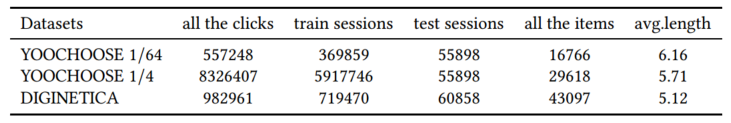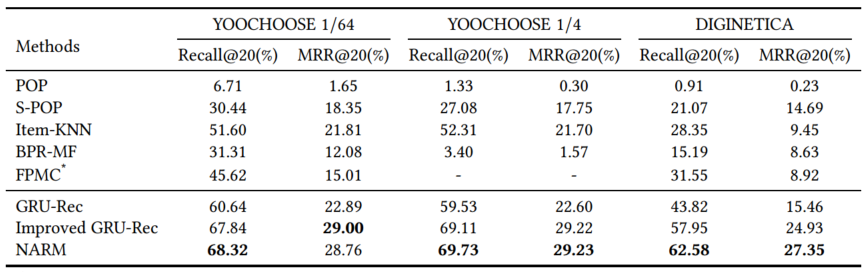Notes: The authors emphasize that in traditional methods, the main purpose of a session was outlooked. And then the authors utilize attention mechanisms to solve this problem. However, at present, using $h_t^g$ as a query is not reasonable enough for three reasons as follows:

1. $h_t^g$ is generated by capture information of the global sequence. So $h_t^g$ is similar to every item in the session, Using it to estimate attention weight tends to still capture the overall info of the sequence.
2. Based on RNN mechanism, $h_t^g$ tends to focus more on the last item. If the last item is not similar as the target item, using $h_t^g$ as query will generate the wrong signal.
3. Based on RNN mechanism, $h_t^g$ tends to focus more on a category of items that are frequently interacted at the sequence. When the user wants to select an item from a lot of categories of items, then $h_t^g$ is about evenly influenced by every category of items and thus still can not capture the main purpose as well as enough.

A possible way to avoid these problems is to use the embedding of the candidate item $i$ as the query to extract the most related preference, denoted as $c_t^i$, of the user for the candidate item. And then the predicted score for each candidate item is calculated as $S_i = emb_i^TBc_t^i$

## Other works that follow the work

### Short-Term Atention/Memory Priority Model for Session-based Recommendation

KDD’18

Gap: Capture both long-term and short-term interests in a session

Method: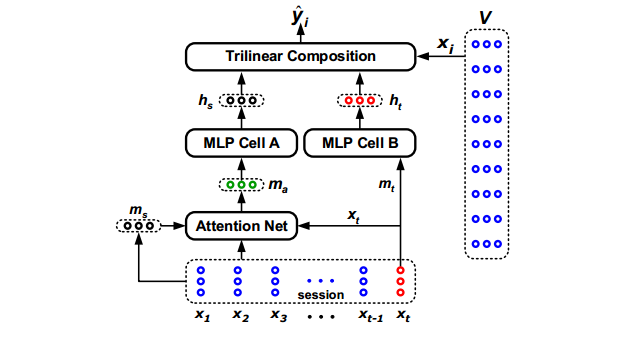As shown in the above figure, given the embeddings ${x_1, x_2,…,x_t}$ of a session, the process is summarized as follows:

1. $m_s$ is the average of all embeddings, and then the long-term interests is got by an Attention Net in which the queries are $m_s$ and $x_t$. Then $m_a$ is transformed to $h_s$ by a MLP layer

2. short-term interest $m_t = x_t$ and then is transformed to $h_t$ by another MLP layer.

3. the score for a candidate item $x_i$ is calculated by a by a trilinear product of three vector defined as:
$$<a, b, c> = \sum_{i=1}^da_ib_ic_i$$

Notes:

1. the model does not use the RNN layer to handle embeddings but directly applies the Attention Network. This may suggest that RNN layer is not important in this work.
2. Also the last embedding is used as query

### Session-based Recommendation with Graph Neural Networks

AAAI’19

Problem: Session Recommendation

Gap: Transitions among distant items are often overlooked by previous methods.

Method: Use Graph to model item relation in sessions and utilize Graph Neural Networks to capturing transitions of items and generate accurate item embedding vectors. For example, as follows, the three sessions are constructed as a graph.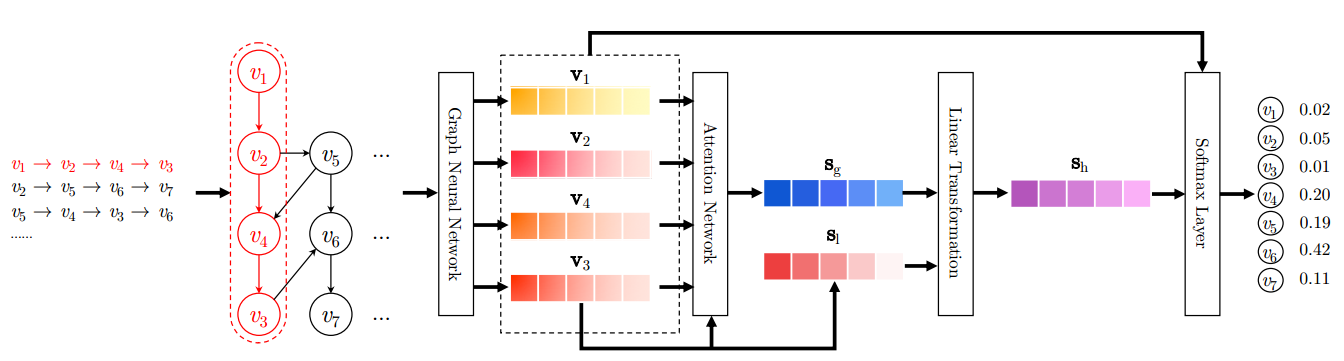Notes:

1. In Attention Network, the authors also use the embedding of the last interacted item in the session as the query.
2. The model also abandoned RNN layer.

### A Repeat Aware Neural Recommendation Machine for Session-based Recommendation

AAAI’19

Problem: session recommendation

Gap: propose a novel repeat consumption phenomenon where the same item is re-consumed repeatedly over time. The following table shows the repeat ratio in three public datasets. However, no previous works have emphasized repeat consumption with neural networks. (Multi-armed bandit problem has been studied for a long time to solve the repeat-explore dilemma for general recommendations.)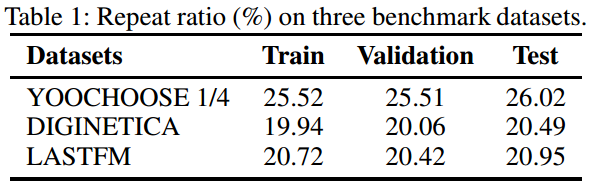Method: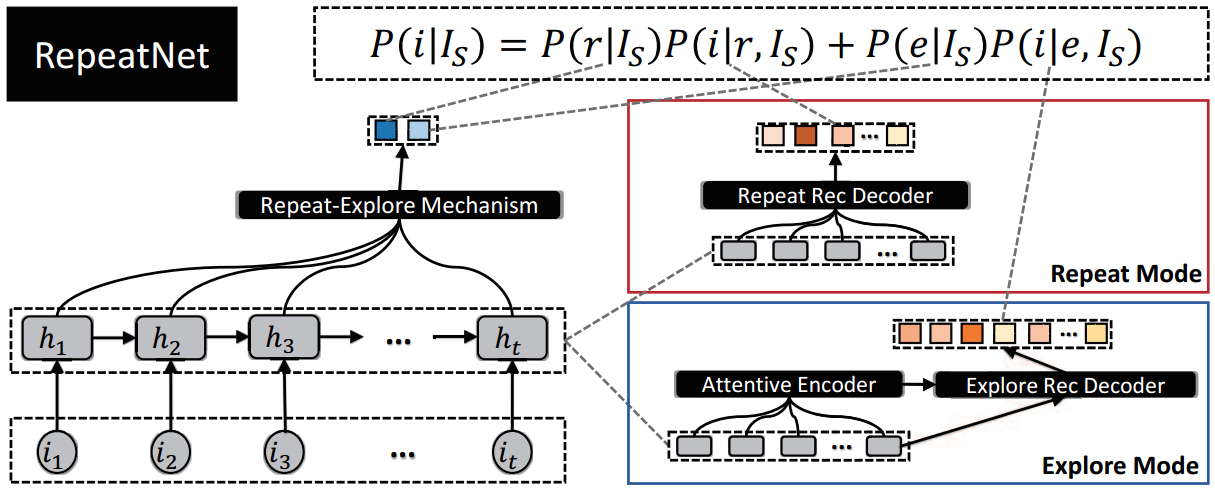As shown in the above figure, the process is summarized as:

1. Use GRU to encode the session sequence of a user.
2. Use attention mechanism to merge all hidden states with the last hidden state as the query. Then use a dense network to transform the merged vector into a two-dimension vector with the first value as the probability of repeat and the other as the probability of explore
3. For repeat mode, decode the hidden states to calculate the score for each item (new items get 0 points)
4. For explore mode, decode the hidden states with attention to calculate for each item (old items get 0 points)
5. Use the score and whether the item is new as supervising info to train the model with a negative log-likelihood loss and a logistic loss.

Notes: In Explore Mode, the Attentive Encoder uses same attention mechanism that uses the last hidden state $h_t$ as the query.

## Attention with Candidate Item

The idea of using the candidate item as the query has been utilized in some previous works. Here are some works.

### Neural Attentive Item Similarity Model for Recommendation

IEEE TRANSACTIONS ON KNOWLEDGE AND DATA ENGINEERING

Problem: CF-based recommendation

Gap: Traditional MF based methods need to retrain for real-time recommendations to update users’ preference vectors. On the other hand, item-to-item CF could make real-time recommendations much easier to achieve. However, previous works ignore different item has a different influence on the recommendation to users. So the authors use attention mechanisms to calculate the weight of influence for each item in one’s historical interactions.

Method: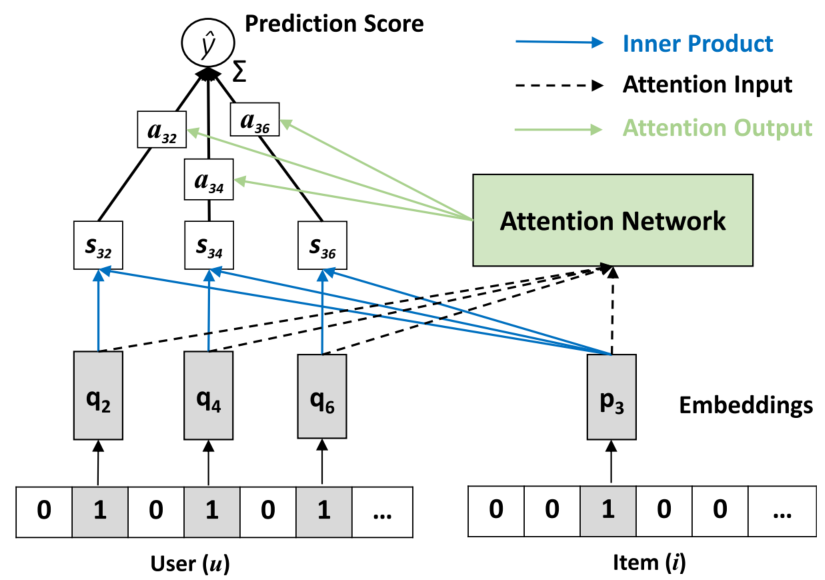As shown in the above figure, the process is summarized as follows:

1. Each item has two embeddings: the embedding, denoted as $q$, when the item is in one user’s interaction and the embedding, denoted as $p$, when the item is a candidate item.
2. The score $s_{ij}$ is the similarity between the item $i$ in the user’s interactions and the candidate item $j$
3. The final score is the weighted sum of all ${s_{ij}}$
4. The weight $a_{ij}$ is estimated by attention mechanism with the candidate item as the query.

Notes: The model ignores the time order of each interacted item. Besides, each item in one’s interactions is independent and thus the context info is neglected.

## Non-attention works

### Improved Recurrent Neural Networks for Session-based Recommendations

Deep learning workshop on RecSys’16

experiments skills, adapt various techniques from the literature for this task.

1. Data augmentation via sequence preprocessing and embedding dropout to enhance training and reduce overfitting
2. Model pre-training to account for temporal shifts in the data distribution
3. Distillation using privileged information to learn from small datasets.

## Some Intuition of Using Candidate Item as Query

### Introduction

From the view of method, we argue that using the last hidden state as the query has the following drawbacks:

1. $h_t^g$ is generated by capture information of the global sequence. So $h_t^g$ is similar to every item in the session, Using it to estimate attention weight tends to still capture the overall info of the sequence.
2. Based on RNN mechanism, $h_t^g$ tends to focus more on the last item. If the last item is not similar as the target item, using $h_t^g$ as the query will generate the wrong signal.
3. Based on RNN mechanism, $h_t^g$ tends to focus more on a category of items that are frequently interacted at the sequence. When the user wants to select an item from a lot of categories of items, then $h_t^g$ is about evenly influenced by every category of items and thus still can not capture the main purpose as well as enough.

For the second drawback, the intuition is obvious. The last interacted item will be not consistent with the next item when the user’s interests drift. Here, we don’t use interest drift to explain this phenomenon. The interests drift is not proper for the phenomenon. We can combine this problem with the first and third drawbacks to define the problem as multi-interests problem: Each user have many kinds of interests.

For example: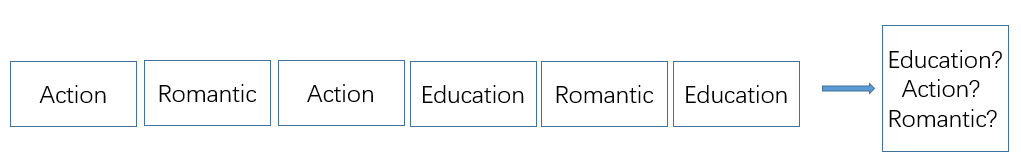As shown in the above figure, from the interaction sequence, we can see the use likes three kinds of movies: Action, Romantic and Education. For the user, the choice of the next movie is mainly dependent on which kind of movie is published or recommended. In this scenario, we can recommend Education, Action or Romantic movies. It’s not proper for the user just like Education movies at present.

To model the interests of users at each time and capture users’ multi-interests at recommendation time, we propose to use RNN to model users’ dynamic interests and the attention mechanism with candidate item as the query to capture the multi-interests.

For the example above, if the candidate item is an Education movie, we will focus more on the forth and the last movies to give the evaluate the score. And the recommendation for Action movies or Romantic movies is similar. Besides, other kinds of movies will get lower attention from all interactions. In this scenario, if we use the last hidden state as the query, then just Education movies will get more attention. Romantic and Action movies may be regarded as noises.

### Support of Multi-interests.

An analysis of course recommendation datasets. (see Hierarchical Reinforcement Learning for Course Recommendation in MOOCs for detail)

For previous attention-based works, when a user has interests in many different courses, the attention mechanism will perform poorly as the effects of the contributing courses are diluted by diverse historical courses. An example is shown in the following figure, for a trained attention-based model, the real target course gets lower recommendation probability than a randomly selected course although it gives more related courses more weights.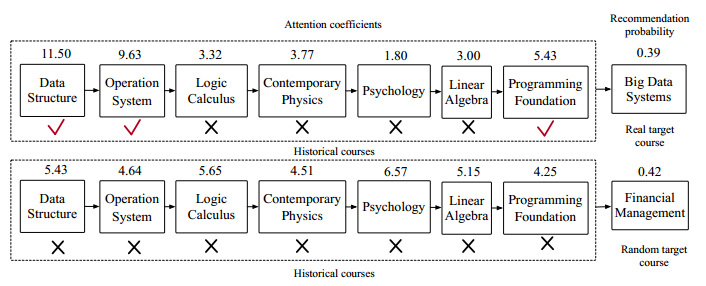More analyses on the dataset are shown in the following figure. From figure (b), we can the category ratio is evenly distributed for all users. From figure (c), we can see as the category ratio grows larger which means these users like more kinds of courses, a trained attention-based model give lower recommendation probability to the target courses.